TitleCollege Algebra
Answer/Discussion to Practice Problems
Tutorial 40: Graphs of Rational FunctionsAnswer/Discussion to 1a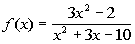Our restriction here is that the denominator of a fraction can never be equal to 0.  So to find our domain, we want to set the denominator equal to 0 and restrict those values.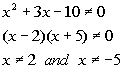*The den. cannot equal to 0 *Factoring to help "solve" *2 and -5 are restricted values

 Our domain is all real numbers except 2 and -5, because 2 and -5 both make the denominator equal to 0, which would not give us a real number answer for our function.Answer/Discussion to 2a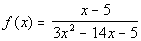Reducing the rational function to lowest terms we get: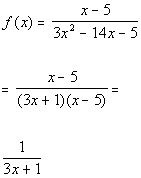*Factor *Cancel the common factor of x - 5

 Vertical Asymptote: So now we want to find where the denominator is equal to 0: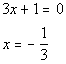*Set den = 0

 There is one vertical asymptote: x = -1/3.

 Horizontal Asymptote: So now we want to compare the degrees of the numerator and the denominator.  What is the degree of the numerator that is left?  If you said 0, you are correct.  The leading term is the constant 1 and its degree is 0. What is the degree of the denominator that is left?  If you said 1, you are correct.  The leading term is x and its degree is 1. If you need a review of finding the degree of a polynomial, feel free to go to Tutorial 6: Polynomials. Since the degree of the numerator is 1 is less than the degree of the denominator, then there is a  horizontal asymptote at y = 0.Answer/Discussion to 2b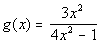This rational function is already in lowest terms: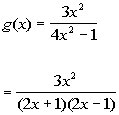Vertical Asymptote: So now we want to find where the denominator is equal to 0: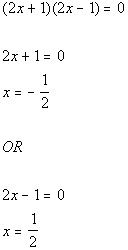*Set den = 0

 There are two vertical asymptotes: x = -1/2 and 1/2.

 Horizontal Asymptote: So now we want to compare the degrees of the numerator and the denominator.  What is the degree of the numerator?  If you said 2, you are correct.  The leading term is the constant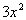and its degree is 2. What is the degree of the denominator?  If you said 2, you are correct.  The leading term is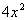and its degree is 2. If you need a review of finding the degree of a polynomial, feel free to go to Tutorial 6: Polynomials. Since the degree of the numerator is equal to the degree of the denominator, then there is a  horizontal asymptote at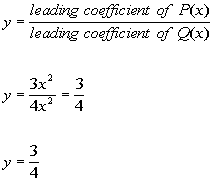Answer/Discussion to 3a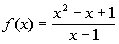Note that this rational function is already reduced down. Applying long division to this problem we get: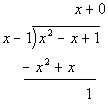The answer to the long division would be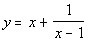. The equation for the slant asymptote is the quotient part of the answer which would be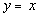.Answer/Discussion to 4a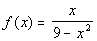Step 1: Reduce the rational function to lowest terms and check for any open holes in the graph.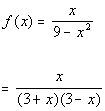*Factor

 This function cannot be reduced any further.  This means that there will be no open holes on this graph.

 Step 2: Find all of the asymptotes and draw them as dashed lines.

 Vertical Asymptote: So now we want to find where the denominator is equal to 0: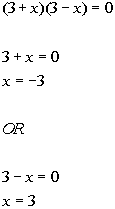*Set den = 0 *Set 1st factor = 0 *Set 2nd factor = 0

 There are two vertical asymptotes: x = -3 and x = 3.

 Horizontal Asymptote: So now we want to compare the degrees of the numerator and the denominator.  What is the degree of the numerator?  If you said 1, you are correct.  The leading term is x and its degree is 1. What is the degree of the denominator?  If you said 2, you are correct.  The leading term is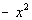and its degree is 2. If you need a review of finding the degree of a polynomial, feel free to go to Tutorial 6: Polynomials. Since the degree of the numerator is 1 is less than the degree of the denominator, then there is a  horizontal asymptote at y = 0.

 Slant Asymptote: Since the degree of the numerator is NOT one degree higher than the degree of the denominator, there is not slant asymptote.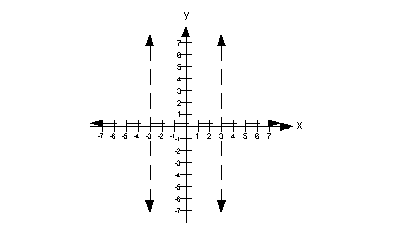Step 3: Determine the symmetry.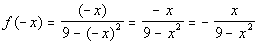Since f(-x) = -f(x) , the function is odd.  This means the graph is symmetric about the origin. If you need a review of even and odd functions, feel free to go to Tutorial 32: Graphs of Functions Part II.

 Step 4: Find and plot any intercepts that exist.

 x-intercept: What value are we going to use for y?  You are correct if you said 0.*Plug in 0 for y *x-intercept at 0

 There is an  x-intercept at (0, 0).

 y-intercept: What value are we going to use for x?  You are correct if you said 0.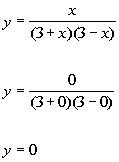*Plug in 0 for x *y-intercept at 0

 There is an  y-intercept at (0, 0).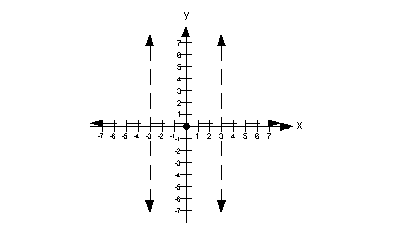Step 5: Find and plot several other points on the graph.

 Note how the vertical asymptotes sections the graph into three parts.  I’m going to plug in two x values that are to the left of x = -3, two in between x = -3 and x = 3, and two that are to the right of x = 3.

 Plugging in -5 for x we get: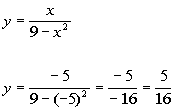(-5, 5/16)

 Plugging in -4 for x we get: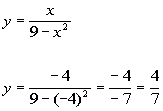(-4, 4/7)

 Plugging in -1 for x we get: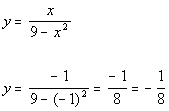(-1, -1/8)

 Plugging in 1 for x we get: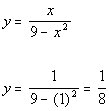(1, 1/8)

 Plugging in 4 for x we get: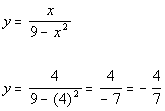(4, -4/7)

 Plugging in 5 for x we get: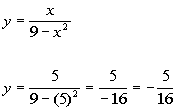(5, -5/16)

 Step 6: Draw curves through the points, approaching the asymptotes.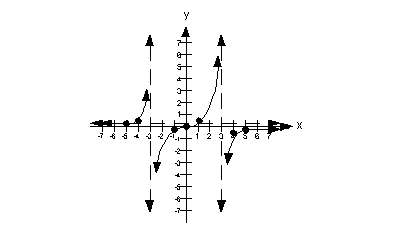Answer/Discussion to 4b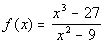Step 1: Reduce the rational function to lowest terms and check for any open holes in the graph.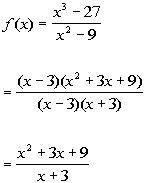*Factor *Cancel the common factor of x - 3

 The factor of x - 3 canceled out and there were no other factors of x - 3 left in the denominator.  This means there is an open hole on the graph at x = 3. x = 3: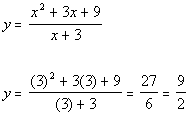*Plug in a 3 for x

 There is an open hole at (3, 9/2).

 Step 2: Find all of the asymptotes and draw them as dashed lines.

 Vertical Asymptote: So now we want to find where the new denominator is equal to 0: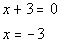*Set den. = 0

 There is one vertical asymptote: x = -3.

 Horizontal Asymptote: Since the degree of the numerator is one degree higher than the degree of the denominator, there is a slant asymptote and no horizontal asymptote.

 Slant Asymptote: Applying long division to this problem we get: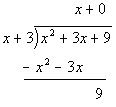The answer to the long division would be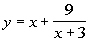. The equation for the slant asymptote is the quotient part of the answer which would be.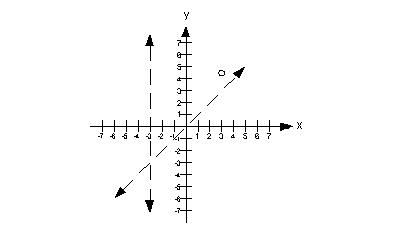Step 3: Determine the symmetry.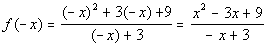Since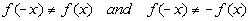, the function is neither even nor odd.  If you need a review of even and odd functions, feel free to go to Tutorial 32: Graphs of Functions Part II.

 Step 4: Find and plot any intercepts that exist.

 x-intercept: What value are we going to use for y?  You are correct if you said 0.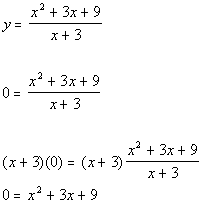*Plug in 0 for y *Mult. both side by the LCD (x + 3) *Does NOT Factor

 There are no real number solutions to this equation, so there are no x-intercepts.

 y-intercept: What value are we going to use for x?  You are correct if you said 0.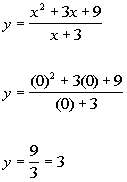*Plug in 0 for x *y-intercept of 3

 The y-intercept is (0, 3).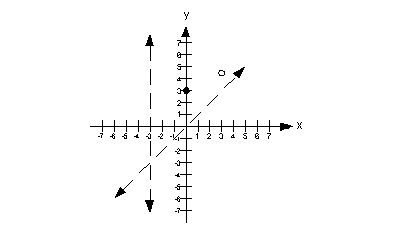Step 5: Find and plot several other points on the graph.

 Note how the vertical asymptote sections the graph into two parts.  I’m going to plug in two x values that are to the left of x = -3 and two x values that are to the right of x = -3 .

 Plugging in -5 for x we get: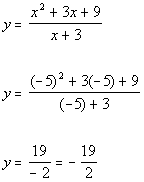(-5, -19/2)

 Plugging in -4 for x we get: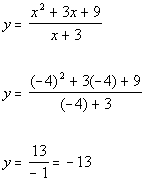(-4, -13)

 Plugging in -2 for x we get: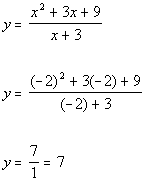(-2, 7)

 Plugging in -1 for x we get: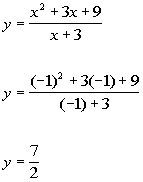(-1, 7/2)

 Step 6: Draw curves through the points, approaching the asymptotes.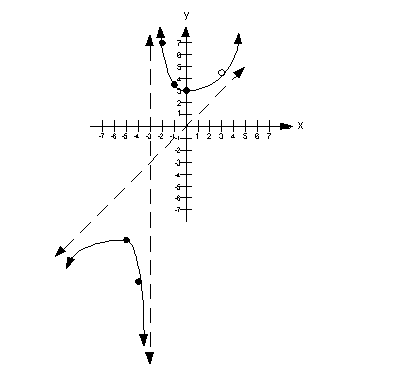Last revised on March 17, 2011 by Kim Seward.
All contents copyright (C) 2002 - 2011, WTAMU and Kim Seward. All rights reserved.